# How to Extract Text Before or After Character in Excel and Google Sheets

This tutorial will demonstrate how to extract text before or after a character in Excel and Google Sheets.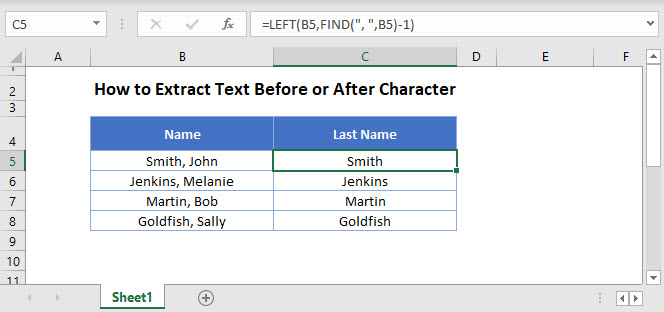## Extract Text Before Character using the FIND and LEFT Functions

To extract the text before the comma, we can use the LEFT and FIND functions

### Find Function

First, we can find the position of comma by using the FIND function and then subtract one to the value returned to get the length of the Last Name.

``=FIND(",", B3)-1``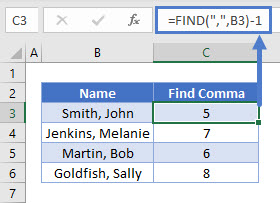### Use LEFT Function

We then use the left function to extract the text before the position returned by the FIND function above.

``=LEFT(B3, C3)``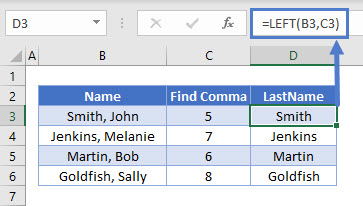Combining these functions yields the formula:

``=LEFT(B3, FIND(",", B3)-1)``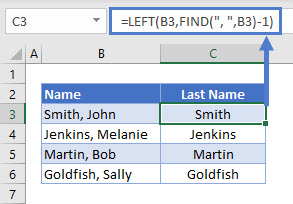## Extract Text After Character using the FIND, LEN and RIGHT Functions

In the next section, we will use the FIND, LEN and RIGHT Functions to extract the text after a specific character in a text string.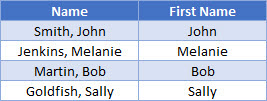### FIND Function

As we did in the previous example, we use the find Function to find the position of the comma and then subtract one to the value returned to get the length of the Last Name.

``=FIND(",", B3)-1``### LEN Function

We then use the LEN Function to get the total length of the text

``=LEN(B3)``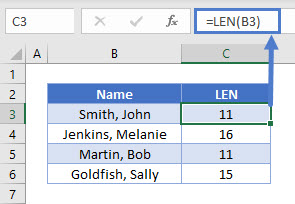We can then combine the FIND and the LEN functions to get the amount of characters we want to extract after the comma

``=LEN(B3)-FIND(",",B3)-1``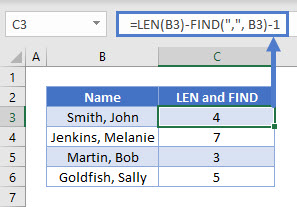### Easy Excel Automation### RIGHT Function

Finally we use the RIGHT function to return the characters after the comma in the cell.

``=RIGHT(B3,C3)``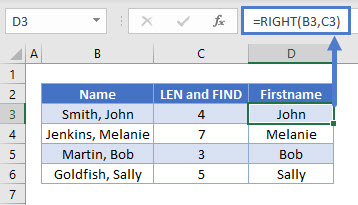Combining these functions yields this formula:

``=RIGHT(B3,LEN(B3)-FIND(",",B3)-1)``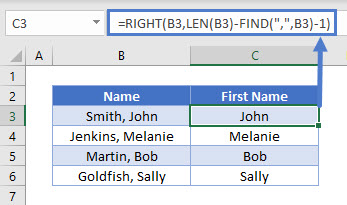## Extract Text Before Character using the FIND and LEFT Functions in Google Sheets

You can extract text before a character in Google sheets the same way you would do so in Excel.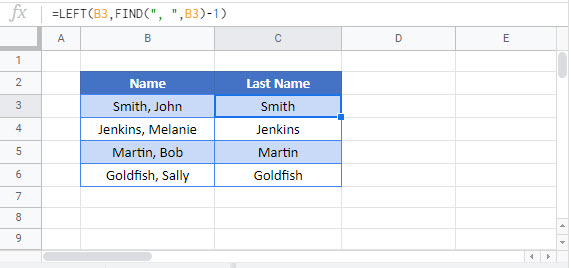## Extract Text After Character using the FIND, LEN and RIGHT Functions in Google Sheets

Similarly, to extract text after a character is also the same in Google Sheets.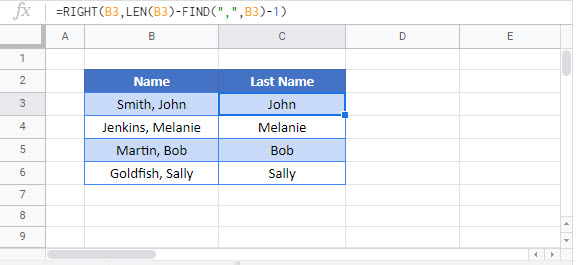### Excel Practice Worksheet

Practice Excel functions and formulas with our 100% free practice worksheets!